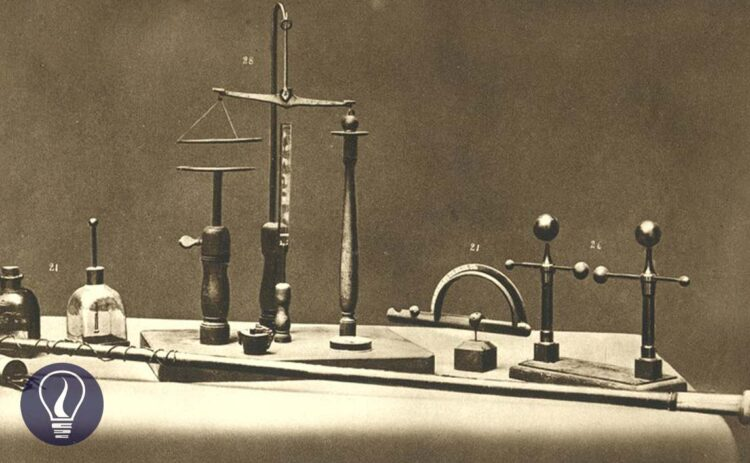More results...

Generic selectors
Exact matches only
Search in title
Search in content
Post Type Selectors

# Static Electric Field (Electrostatic Field)B.Sc (Hons, USJ) (Polymer Science and Technology, Chemistry, Physics)
Categories
Last Updated On : September 20, 2023
Published Date : September 20, 2023

## What is a static electric field?

A static electric field also known as an electrostatic field is a region that can affect the static electric charges without any contact or gravitational field. An electric field is generated due to two types of electrostatic charges. Those are positive charges and negative charges. The smallest possible negative charge is the electron. And the smallest possible positive charge is the proton.

Charge of electron = – 1.60217663 × 10-19 C

Charge of proton = +1.60217663 × 10-19 C

Generally, objects are neutral. That means there are equal amounts of negative and positive charges in the object. When objects are charged, only electrons can be removed or added to the object. If the electrons are removed from the object, the object becomes positively charged. The object becomes negatively charged if the electrons are added to the object.

We can find the charge of any positively charged object as follows.

Where,

• Q = Charge of the object
• n = Number of removed electrons
• e = charge of the electron

We can find the charge of any negatively charged object as follows.

Where,

• Q = Charge of the object
• n = Number of added electrons
• e = charge of the electron

The SI unit of the electric charge is Coulomb (C).

## Methods of imparting a static electric charge to an object

There are three methods by which an object can be given a static electric charge. They are

1. Rubbing method - By friction
2. Contact method
3. Induction

### Rubbing method

When rubbing two objects, due to the friction, electrons are removed from one object and added to the other object.

E.g.

• When an ebonite rod is rubbed with a wool cloth, electrons are removed from the wool cloth and added to the ebonite rod. Here, the ebonite rod becomes negatively charged and the wool cloth becomes positively charged.
• When a glass rod is rubbed with a silk cloth, electrons are removed from the glass rod and added to the silk cloth. Here, the glass rod becomes positively charged and the silk cloth becomes negatively charged.

Objects with similar charges repel each other and objects with opposite charges attract each other. (The Coulomb Force)

### Contact method

We can charge only electrically conductive materials by the contact method. When two electrically conductive objects with charge touch each other, electrons move moving one object to another until the electric potential becomes the same. If the two objects are identical, charges are shared equally.

Example 01 - Calculating the electric charge of an object

There are two conductive balls A and B. The electrical charges of the two balls are -97 e and +3 e. A and B are touched together instantly and separated. If A and B are identical what is the charge of each ball?

Solution

## How to find if there is a static electric charge in an object

We can find whether there is a charge in an object by a pith ball experiment or using a gold leaf electroscope.

### Pith ball experiment

Pith balls are small, lightweight balls (mostly made out of Styrofoam) that are coated with a conductive coating. A pith ball which is freely hanging by an insulating thread approaches an object, if the pith ball attracts to the object, the object has a charge.

If the pith ball is attracted to the object and repels again after contact, then the object is a conductor. If the pith ball remains attracted to the object after contact, the object is an insulator.

#### How to determine the type of charge of an object using a pith ball

1. First, an ebonite rod is rubbed with a wool cloth. Here, the ebonite rod becomes a negatively charged object.
2. Then the ebonite rod is brought closer to the neutral pith ball.
3. Without removing the ebonite rod, the pith ball is grounded. The pith ball can be grounded by touching it by hand.
4. Without removing the ebonite rod, the grounded connection is disconnected.
5. Then the ebonite rod is removed. Now the pith ball has become a positively charged object.
6. When an object is brought closer to this pith ball, if the ball repels that means the object has the same charge that the pith ball does. (Positive charges). If the pith ball attracts the object, that means the object has the opposite charge that the pith ball does (Negative charges).## References and Attributes

### Figures:

The cover image was designed using an image by Dr.Antonio Carlos Mde Queiroz, licensed under Creative Commons Attribution-Share Alike 4.0 International, via Wikimedia Commons